Trial ends in

# Investigation Ohm's Law for Ohmic and Nonohmic Conductors

### Overview

Source: Andrew Duffy, PhD, Department of Physics, Boston University, Boston, MA

This experiment investigates Ohm's law, which relates current, voltage, and resistance.

One goal of the experiment is to become familiar with circuit diagrams and the terminology involved in basic circuits, such as resistor, resistance, current, voltage, and power supply. By the end of the experiment, familiarity is gained with how to wire up a circuit and how to measure both the current passing through a circuit component and the potential difference, or voltage, across it.

In a circuit, a battery or power supply provides a voltage measured in volts (V) that makes the charge flow. Other elements in the circuit, such as light bulbs or resistors (which are often just long narrow wires wound into coils) limit the rate at which the charge flows. The rate of flow of the charge is known as current measured in amperes (A), or amps for short, and the degree to which resistors and light bulb filaments limit the flow is known as their resistance measured in ohms (Ω). This experiment involves an exploration of Ohm's law, which relates voltage, current, and resistance.

This experiment also explores the difference between a basic circuit component called a resistor, and a light bulb and a light-emitting diode (LED). Light bulbs and LEDs are part of many common devices and are used for various lighting applications, and so it is useful to understand how they work.

### Principles

Ohm's law states that the current through a device is directly proportional to the potential difference applied to the device. Ohm's law can be applied to individual circuit components, or to a circuit as a whole, to predict values of current, if voltage and resistance are known, or to determine the resistance, if voltage and current are measured.

Resistance is a measure of how difficult it is for charge to flow. Some resistors have a resistance that is approximately constant, while in others, the temperature dependence of resistance is an important factor. Note that resistance increases, in general, as temperature increases. A diode is essentially a one-way valve for charge, allowing current to flow easily in one direction, but not allowing much current to flow in the opposite direction. The resistance of a diode is strongly dependent on the voltage across it, and this will be demonstrated in the experiment.

The data collected in the experiment will be used to study Ohm's law in various circuits.

### Procedure

1. Becoming Familiar with the Apparatus

1. For this experiment, use a computer to collect the data and plot graphs.
2. Load the software for the experiment by double-clicking on the "Ohm's Law" icon on the computer desktop. When the program loads, the screen should show a graph, a table, and in the lower right corner, boxes with the voltage and current readings.
3. Click the Zero button and select "Zero All Sensors" to make sure that the voltage and current readings read zero when they are unconnected.
4. Following the circuit diagram in Figure 1, connect the two leads on the voltage sensor to the power supply, which is a blue box. The red lead goes to the + connection on the power supply, and the black lead goes to the - one.
5. Make sure that the current sensor is not connected, and ensure that the current knob on the power supply is turned to maximum (fully clockwise).
6. Start the data collection by pressing the green arrow icon (the Collect button) on the computer.
7. Observe what happens when the voltage knob on the power supply is moved between its minimum and maximum settings. Adjusting the knob allows the voltage to be changed from about +0.7 V to at least +5 volts.
8. Reverse the leads of the voltage sensor (red to -, black to +), and repeat the steps 1.5-1.7. This time, adjusting the knob gives a voltage range of about −0.7 V to -5 V. Thus, by adjusting the power supply voltage as well as reversing the leads, almost the full range of voltages between -5 V and +5 V is available to work with in this experiment. Note that the power supply used in this experiment cannot put out voltages below 0.7 volts (an unusual feature), but there is more than enough of a range to determine the relationships of interest.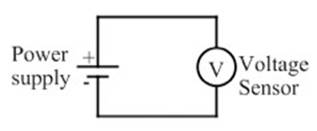Figure 1: This circuit diagram shows the power supply (marked with the + and - symbols) and the voltage sensor (V) connected by two wires.

2. Investigating Ohm's Law

Note: In this part of the experiment, the goal is to observe graphs of current vs. voltage.

1. To change what is displayed on an axis, click on the axis label, check the appropriate option to display the desired information, and (for the y-axis only) remove the check(s) for unwanted items on the display. Set the graph to show current on the y-axis and potential on the x-axis.
2. To set the scales properly, click on one of the numbers to the left of the y-axis. This brings up a box to enter a minimum of -0.3 and a maximum of 0.3 for the manual scaling. Repeat for the x-axis by clicking on a number below the x-axis to bring up the settings box. This time, enter -6 as the minimum and 6 as the maximum. This prepares the graph to display currents between −0.3 and +0.3 A, and voltages between -5 V and +5 V.
3. Following the circuit diagram in Figure 2, wire the circuit to measure the voltage and current across a 100 Ω resistor.
4. Use a resistance box as the resistor. Set it for 100 Ω. On the power supply, turn both the current and the voltage to maximum.
5. Press the green arrow icon to start the data collection.
6. Over several seconds, turn the voltage down to minimum, then quickly reverse the leads at the power supply. Over several seconds, turn the voltage knob toward maximum again. This should result in a graph of current vs. voltage, ranging from about -5 volts to +5 volts.
7. Repeat the process until satisfied with the graph, select the Data menu, and choose "Store Latest Run."
8. Observe that the graph of current vs. voltage is a straight line. Hit the Regression button to perform a linear fit to the data, and record the slope of the line.
1. Figure 4 shows the current vs. voltage graph for the resistor marked 100 Ω. A linear fit to the data results in a slope of 0.00991 A/V. The inverse of the resistance is 0.0100 A/V, which is a good match to the slope.
9. Change the resistance of the resistance box to 200 Ω, and repeat the steps 2.6-2.9.
10. Compare the two slope values to the corresponding resistance values, and determine the relationship between current, voltage, and resistance.
1. Figure 5 shows the current vs. voltage graph for the resistor marked 200 Ω. A linear fit to the data results in a slope of 0.00510 A/V. The inverse of the resistance is 0.00500 A/V, which is a good match to the slope.
11. The slope of the current vs. voltage graph turns out to be the inverse of the resistance. This can be written as: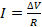or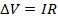1. The current sent out by the power supply is proportional to the voltage and inversely proportional to the resistance. When the current is graphed as a function of the voltage, the slope of the line is expected to be the inverse of the resistance.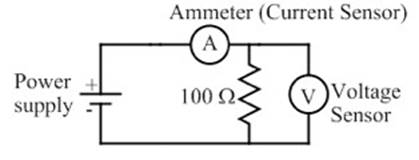Figure 2: This circuit diagram shows the power supply connected to a 100 Ω resistor, with the voltage sensor (V) measuring the potential difference across the resistor and the ammeter measuring the current through it.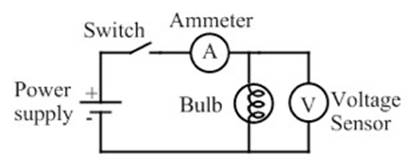Figure 3: In this circuit diagram, the resistor is replaced by a light bulb, and a switch has been added. The switch is initially open, so that the light bulb is off at the start.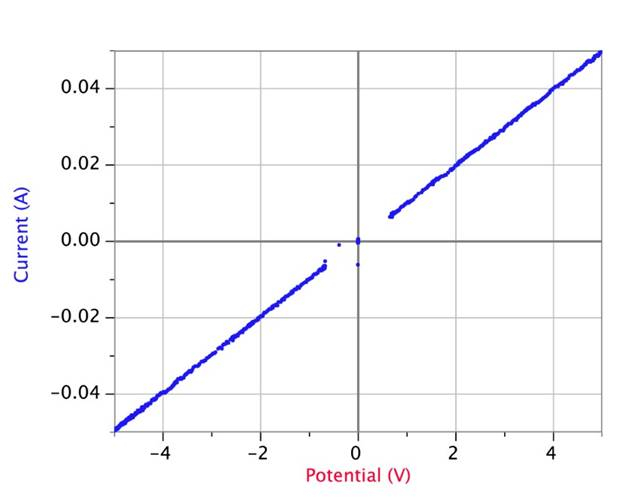Figure 4: Current vs. voltage graph with a resistor marked 100 Ω. A linear fit to the data results in a slope of 0.00991 A/V. Note that the missing data at the center of the graph is simply an artifact of the particular power supply used in the experiment, which has the unusual feature of not producing voltages below about 0.7 V.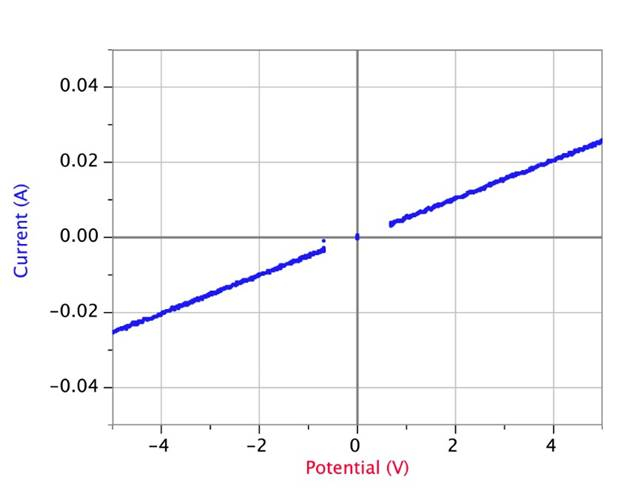Figure 5: Current vs. voltage graph with a resistor marked 200 Ω. A linear fit to the data results in a slope of 0.00510 A/V.

3. Investigating Light Bulbs

1. Replace the resistor from the previous circuit with a small incandescent light bulb ( Figure 3). Repeat the experiment, obtaining a graph of current as a function of voltage for the light bulb. Note any differences in graph form for the light bulb compared to the resistor.
1. Figure 6 shows a graph of current vs. voltage for the incandescent light bulb. In general, the current increases as the voltage increases, but the graph is not nearly as linear as the graphs for the resistors. The graph also shows that the current is, in general, higher at a given voltage when the voltage is increasing, compared to its value at that same voltage when the current is decreasing.
2. Now, plot current vs. time instead of current vs. voltage. To do this, click on the "voltage" label on the horizontal axis to bring up the list of variables that the graph can be plotted against, and choose time instead of voltage.
3. Turn the voltage up, so that the bulb glows brightly, and then switch the power supply off, using the on/off switch. Click on the Collect button (green arrow) on the screen to start the data collection, and switch the power supply back on.
1. Observe the graph, shown in Figure 7. The current through the light bulb goes to a high level when the power supply is switched on, and then drops to a smaller constant value. This is quite different than the behavior of a standard resistor, which would typically jump straight to a constant value when the power supply is turned on.
2. The explanation for both of these observations is the same. The higher the temperature of the light bulb filament, the larger the resistance. When the bulb is off ( Figure 7), the filament is at room temperature with a relatively low resistance. When the bulb is then turned on, the current jumps to a high level because of the low resistance, but as the filament heats up and the resistance increases, the current drops. Eventually, the temperature stabilizes, and the current is constant.
3. When the voltage is increasing ( Figure 6), the filament is warming up, so with a cooler filament the resistance is lower and the current higher. For the second half of the graph, when the voltage reduces, the filament is cooling down from a higher temperature, and thus has a higher resistance and lower current.
4. Finally, switch back to current vs. voltage, and use a LED in place of the light bulb.
5. As before, obtain a graph of current as a function of voltage for the LED. Be sure to use both positive and negative voltages to see the full range of the circuit behavior with the LED.
1. Figure 8 shows the current vs. voltage graph for a LED. The diode does not allow current to flow in one direction, when the voltage is negative; however, when the voltage is positive and above a certain threshold, current flows and increases as quickly as voltage increases. In this way, the diode acts as a one-way valve for current. Note that the light-bulb filament and the resistors show no such directionality.Figure 6: Current vs. voltage graph for an incandescent light bulb. The graph starts at the bottom left, then followed the upper track as the voltage was increased, and the bulb became very bright. The voltage was then decreased, and the graph followed the lower track back down to the bottom left.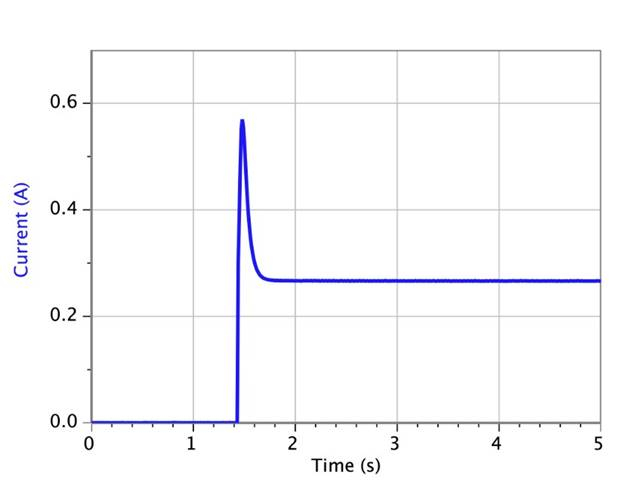Figure 7: Current vs. time graph for an incandescent light bulb. The bulb was off, and then the voltage was turned on at around the 1.4 second mark and kept at a constant value. The current peaked at about 0.57 A, and then dropped down to a constant value of about 0.27 A.Figure 8: Current vs. voltage graph for a diode. A diode, in some sense, acts as a one-way valve for current. The diode does not allow current to flow when the voltage is negative, but when the voltage is positive and above a certain threshold, the current flows and increases as quickly as voltage increases in one direction.

Ohm's law relates voltage, current, and resistance for an electrical component, or a circuit.

Voltage, V, current, I, and resistance, R, are fundamental to the operation of all electronic devices like radios, music players and computers as well as simpler electrical applications like house wiring, fuse boxes and home lighting. The circuits in all of these cases behave predictably and can be designed rationally because of Ohm's law.

This video will introduce circuit terminology, symbols and diagrams, and then demonstrate how to wire a simple circuit. In addition, the current through a component and the voltage across a component will be measured.

The output of a power supply or battery has both positive and negative terminals, which respectively define high and low values of a quantity called electric potential. The difference in this electric potential is voltage, measured in volts. To learn more about these terminologies, please see the video on electric potential in this collection.

A circuit is a network of components connected together to perform a particular function. Current is the movement of a quantity of charge per second and is measured in amperes, or amps, for short. Interestingly, only electrons, which have negative charge, move through wires in a circuit. Because of their negative charge, electrons flow in the direction opposite to that of current. Electrical current can only flow through wires and components connected in a complete loop, much like water current from a reservoir through a pump, into a water wheel and back into the reservoir.

To some degree all electrical elements impede the flow of current, like the bottleneck in a pipe that reduces the flow of water. Resistance describes this phenomenon, and is measured in ohms. Ohm's law defines resistance as voltage across a component divided by current through the component.

For components specifically called resistors, the resistance is approximately constant. The resistor on a common circuit board is typically a small cylindrical object with bands representing a color code for the resistance. By Ohm's law, the current through a constant resistance is directly proportional to the applied voltage and inversely proportional to resistance. In reality, the resistance of most materials generally increases as temperature increases.

The resistance of some devices, like diodes, also varies with the operating condition-that is, voltage and current-as well as other factors. A diode is a device that, to a very good approximation, allows current to flow in one direction only. As a result, it behaves as a one-way valve, passing current through a very low resistance in the "forward" direction and prohibiting current with an extremely high resistance in "reverse."

A light emitting diode, also known as an "LED", is a diode that is illuminated with the flow of forward current. Like a simple diode, an LED does not pass current in the reverse direction, in which case it is not illuminated.

With a simple relationship among voltage, current and resistance, Ohm's law-often expressed as V equals I times R-makes it possible to calculate any one of these quantities if the other two are known.

This video will show that the voltage across a component and the current through it may be easily measured. These experiments will also demonstrate Ohm's law in various circuits and illustrate the relationship between current and voltage for a resistor, a light bulb, and a light emitting diode.

The measurement apparatus consists of a voltage sensor, a current sensor, a power supply, a computer controlled measurement system, and the components to be tested.

To load the measurement software, double-click on the "Ohm's Law" icon on the computer desktop. After the program loads, the screen should display a graph, a table and in the lower left corner, boxes with the voltage and current measurements.

Click the "Zero" button and select "Zero All Sensors" to remove offsets in the data acquisition system. Voltage and current readings should be zero when the leads to the apparatus are not connected to anything.

Select the data that will be plotted by clicking on the axis label and checking the desired option. For the y-axis only, uncheck items that will not be plotted. Set the graph to show current on the y-axis and voltage on the x-axis.

Next, set the y-axis scale to negative 0.3 to positive 0.3 amps, and set the x-axis scale to negative 5 to positive 5 volts.

A resistance box, which can be set for different values of resistance, is used in the first experiment in order to observe how current varies with voltage for a resistor.

Set the resistance box to 100 ohms. Then wire together the power supply, voltage and current sensors, and resistance box as shown in the circuit diagram. Finally, set both the current and voltage outputs of the power supply to the maximum values.

Click the green arrow icon to start data collection. Slowly reduce the power supply voltage to its minimum value. Then, reverse the leads at the power supply and slowly increase the voltage back to its maximum value. This should result in a graph of current versus voltage, spanning the range from -5 to +5 volts. Repeat this process until the graph is free of noise, then store the data.

For the 100 ohm resistance, the plot of current versus voltage is a straight line. Perform a linear fit to the data and record the slope of the line. The slope should be very close to 0.0100 amp/volt, the inverse of the resistance.

Now set the resistance box to 200 ohms and repeat the experiment to obtain another plot of current versus voltage over the range of -5 to +5 volts. This time the slope should be very close to 0.00500 amp/volt, again the inverse of the resistance.

For a constant resistance, Ohm's law states that current through the resistor is proportional to the applied voltage and inversely proportional to resistance. This is apparent in the data for both the 100 ohm and 200 ohm resistors.

For the next experiment, replace the resistor box with a small incandescent light bulb, as shown in the schematic diagram. Set the voltage control on the power supply to maximum and begin data collection. Slowly reduce voltage to the minimum value then slowly increase voltage back to the maximum. The computer will display a plot of current versus voltage over the range of about +0.7 to +5 volts.

Reverse the power supply leads, set the voltage control to maximum, and repeat the process of reducing the voltage to the minimum value and increasing it to the maximum value again. The computer will display a plot of current versus voltage over the range of about -0.7 to -5 volts.

The plot of current versus voltage for the light bulb is not nearly as linear as for the resistors. The graph also shows that in general the current is higher at a given voltage when voltage is increasing, compared to what it is at the same voltage when voltage is decreasing.

When the voltage is increasing, the filament is warming up. With a filament that starts cooler, the resistance is lower and the current is higher. When the voltage is decreasing, the filament is cooling down from a higher temperature, so it has a higher resistance and a lower current at the same operating point.

Now plot current versus time instead of current versus voltage. To do this, change the horizontal axis to measure time.

Adjust the voltage to its maximum, so the bulb glows brightly. Then turn off the power supply. Click on the green arrow to start data collection and then turn the power supply back on.

Current through the light bulb is high immediately after the power supply is turned on, then drops to a lower, constant value. While the bulb is off, the filament is at room temperature and has a relatively low resistance.

When the bulb is first turned on, the current jumps to a high level because of that low resistance. However, the resistance of the filament increases significantly with temperature-as the filament heats, resistance grows and current drops. Eventually its temperature stabilizes and current is constant.

Finally, set the axis to display current versus voltage again and use a light-emitting diode-an "LED"-in place of the light bulb. The maximum current for common LED's is around 30 mA, so the current must be carefully monitored to prevent burning out the LED.

Use the procedure from the prior experiments to obtain a graph of current as a function of voltage for the LED. First apply positive voltage across the LED, and adjust the power supply voltage from maximum to minimum. Then switch the power supply leads and adjust the voltage from minimum back to maximum to observe the directionality of the LED.

The resulting plot shows that an LED allows current to flow only when the voltage is positive and greater than a certain threshold. Once the diode "turns on," current increases quickly as voltage increases. However, no current flows for negative voltage. This behavior demonstrates how an LED acts like a one-way valve for current.

Electronic gadgets are omnipresent in today's world, and Ohm's law has a role to play in every single one of these gadgets.

For instance, the bulb in a flashlight is designed to work with two 1.5-volt batteries in series. Therefore, a light bulb with a suitable resistance must be chosen, so that the batteries provide an appropriate amount of current to make the bulb shine brightly, without burning out. Ohm's law helps guide that choice of bulb.

Another application of Ohm's law is to limit the current supplied to a particular device, perhaps to reduce the risk of electric shock, or to protect the device itself. Ohm's law tells us that, for a given voltage, the higher the resistance, the lower the current. Therefore, by placing a resistance in series with the device, we can limit the current flowing through the device and thus prevent any potential damage.

You've just watched JoVE's introduction to Ohm's law. You should now understand the relationship between the voltage across an electrical component, its resistance, and the resulting current through it, as well as the differences in the electrical behavior of resistors, light bulbs, and light emitting diodes. Thanks for watching!

### Applications and Summary

Electronic gadgets are omnipresent in today's world, and Ohm's law has a role to play in each of these gadgets. For instance, a flashlight designed to work on two 1.5-volt batteries in series (a total of 3 volts), must have a light bulb with an appropriate resistance, so that the batteries provide an appropriate amount of current to allow the bulb shine brightly, without burning out. Ohm's law helps guide that choice of bulb.

Another application is a three-way light bulb, which can shine with three different levels of brightness. One way to make such a bulb is to have a single filament, but place the voltage across different parts of it, depending on which brightness is desired. Again, Ohm's law plays a role in determining the fraction of the filament that the the voltage is placed across, and the resistance of the entire filament.

Another application of Ohm's law is to limit the current supplied to a particular device, perhaps to reduce the risk of electric shock, or to protect the device itself (high currents can cause overheating and burning out). Ohm's law tells us that, for a given voltage, the higher the resistance, the lower the current, and so the current can be limited in a device by placing a large resistance in series.X